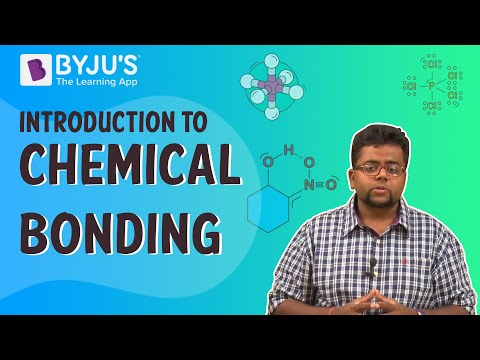Jet Set Go! All about Aeroplanes Jet Set Go! All about Aeroplanes

# Chemical Reaction and Equation MCQ Questions

Chemists use chemical equations to describe chemical reactions, transforming one form of matter (reactants) into another form of matter (products). The unbalanced chemical reaction of methane (CH4) combustion with oxygen (reactants) to produce carbon dioxide and water is shown below (the products).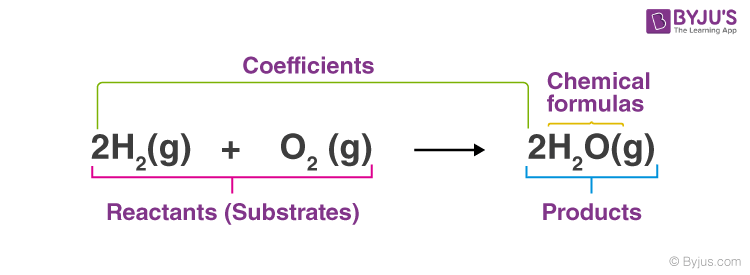Definition: Chemical equations are symbolic expressions of chemical reactions that express the reactants and products in terms of their chemical formulae. Chemical equations also use symbols to depict factors such as the direction of the reaction and the physical states of the reacting entities. In 1615, the French chemist Jean Beguin was the first to establish chemical equations.

## Chemical Reaction and Equation MCQ Chemistry Questions with Solutions

Q1: Magnesium ribbon is rubbed before burning because it has a coating of

(a) basic magnesium carbonate

(b) basic magnesium oxide

(c) basic magnesium sulphide

(d) basic magnesium chloride

Explanation: Magnesium is a heavily reactive metal. It generates a layer of magnesium oxide on its surface when exposed to air, limiting additional magnesium-oxygen reactions. Because it has a covering of basic magnesium oxide, the magnesium ribbon is rubbed before burning.

Q2: Which of the following are exothermic processes?

(i) Reaction of water with quick lime

(ii) Dilution of an acid

(iii) Evaporation of water

(iv) Sublimation of camphor (crystals)

(a) (i) and (ii)

(b) (ii) and (iii)

(c) (i) and (iv)

(d) (ii) and (iv)

Explanation: Calcium hydroxide is produced when water reacts with quick lime, coupled with the release of heat. As a result, the reaction is exothermic. Diluting a concentrated acid is an extremely exothermic process.

CaO + H2O → Ca(OH)2 + Heat

H2SO4 + H2O → H3O+ + HSO4 + Heat

Q3: MnO2 + 4HCl → MnCl2 + 2H2O + Cl2

Identify the substance oxidized in the above equation.

(a) MnCl2

(b) HCl

(c) MnO2

(d) H2O

Explanation: Here HCl is oxidized to Cl2 and MnO2 is reduced to MnCl2.

Q4: A substance ‘X’ is used in white-washing and is obtained by heating limestone in the absence of air. Identify ‘X’.

(a) CaOCl2

(b) Ca(OH)2

(c) CaO

(d) CaCO3

Explanation:

$$\begin{array}{l}CaCO_{3} \overset{Heat}{\rightarrow} CaO + CO_{2}\end{array}$$

Limestone Quick lime

$$\begin{array}{l}CaO + H_{2}O \overset{Heat}{\rightarrow} Ca(OH)_{2}\end{array}$$

Quick lime Slaked lime

$$\begin{array}{l}Ca(OH)_{2} + Cl_{2} \overset{Heat}{\rightarrow} CaOCl_{2} + H_{2}O\end{array}$$

Slaked lime Bleaching powder

Q5: Which of the following is an endothermic process?

(a) Dilution of sulphuric acid

(b) Condensation of water vapours

(c) Sublimation of dry ice

(d) Respiration in human beings

Answer: (c) Sublimation of dry ice

Explanation: Sublimation of ice is an immediate phase transition from solid to gas without having to pass through an intermediate liquid state. Because the process requires additional energy, it is an endothermic reaction, and the heat absorbed is referred to as heat of sublimation.

Q6: Select the oxidising agent for the following reaction:

H2S + I2 → 2HI + S

(a) H2S

(b) I2

(C) HI

(d) S

Explanation: Here, Hydrogen is removed from H₂S [Removal of hydrogen is oxidation]. In addition, H2S is altering in S. H2S is being depleted of hydrogen by I2. As a result, iodine is an oxidising agent.

Q7: What type of chemical reactions take place when electricity is passed through water?

(a) Displacement

(b) Decomposition

(c) Combination

(d) Double displacement

Explanation: Electrolysis of water is a decomposition reaction.

$$\begin{array}{l}2H_{2}O(l) \xrightarrow[]{Current} 2H_{2}(g) + O_{2}(g)\end{array}$$

Q8: The condition produced by aerial oxidation of fats and oils in foods marked by unpleasant smell and taste is called:

(a) antioxidation

(b) reduction

(c) corrosion

(d) rancidity

Explanation: Rancidity is a term used to describe the development of an unpleasant odour and taste in fat and oil-containing foods as a result of aerial oxidation. It refers to the oxidation of fatty and greasy substances.

Q9: A dilute ferrous sulphate solution was gradu¬ally added to the beaker containing acidified permanganate solution. The light purple colour of the solution fades and finally disap¬pears. Which of the following is the correct explanation for the observation?

(a) KMnO4 is an oxidising agent, and it oxidises FeSO4.

(b) FeSO4 acts as an oxidising agent and oxidises KMNO4.

(c) The colour disappears due to dilution; no reaction is involved.

(d) KMnO4 is an unstable compound and decomposes in the presence of FeSO4 to a colourless compound.

Answer: (a) KMnO4 is an oxidising agent, and it oxidises FeSO4.

Explanation: The oxidising agent in this process is potassium permanganate (KMnO4). It oxidises ferrous sulphate to ferric sulphate in the presence of dilute H2SO4.

$$\begin{array}{l}2KMnO_{4} + 10FeSO_{4} + 8H_{2}SO_{4} \to K_{2}SO_{4} + 2MnSO_{4} + 5Fe(SO_{4})_{3} + 8H_{2}O\end{array}$$

Q10: Pb + CuCl2 → PbCl2 + Cu

The above reaction is an example of:

(a) combination

(b) double displacement

(c) decomposition

(d) displacement

Explanation: A displacement reaction is illustrated by the given reaction. A displacement reaction occurs when one element of a compound is replaced by another element of the compound.

Q11: Which of the following gases can be used for storage?

(a) Carbon dioxide or Oxygen

(b) Helium or Nitrogen

(c) Carbon dioxide or Helium

(d) Nitrogen or Oxygen

Explanation: Because helium, as well as nitrogen, prevent the oil from becoming rancid, they can be used to store a fresh sample of oil for a long time. The use of nitrogen or helium as a blanketing gas prevents air and oil contact.

Q12: Which of the following statements about the given reaction are correct?

3Fe (s) + 4H2O (g) → Fe3O4 (s) + 4H2 (g)

(i) Iron metal is getting oxidised

(ii) Water is getting reduced

(iii) Water is acting as reducing agent

(iv) Water is acting as oxidising agent

(a) (i), (ii) and (iii)

(b) (iii) and (iv)

(c) (i), (ii) and (iv)

(d) (ii) and (iv)

Answer: (c) (i), (ii) and (iv)

Explanation: Here, oxygen reacts with water to get oxidised. The amount of oxygen in water is reduced due to the removal of oxygen. Water is an oxidising agent as well as a source of oxygen.

Q13: Oxidation is a process that involves

(c) removal of oxygen

(d) removal of hydrogen

Explanation: The process of oxidation involves the addition of oxygen, the removal of hydrogen, or the loss of electrons.

Q14: Give the ratio in which hydrogen and oxygen are present in water by volume.

(a) 1:2

(b) 1:1

(c) 2:1

(d) 1:8

Explanation: H2O is the chemical symbol for water. Each water molecule has two hydrogen atoms and one oxygen atom. The H2O molecule breaks up into two hydrogen and one oxygen atoms when electricity passes through it.

Q15: Which among the following statement(s) is (are) true?

Exposure of silver chloride to sunlight for a long duration turns grey due to

(i) the formation of silver by decomposition of silver chloride

(ii) sublimation of silver chloride

(iii decomposition of chlorine gas from silver chloride

(iv) oxidation of silver chloride

(a) (i) only

(b) (i) and (iii)

(c) (ii) and (iii)

(d) (iv) only

Explanation: Silver chloride decomposes into silver metal and chlorine gas when exposed to light.

$$\begin{array}{l}2AgCl \xrightarrow[]{Light} 2Ag + Cl_{2}\end{array}$$

Due to the formation of silver metal, the white colour of silver chloride turns grey.

## Practise Questions on Chemical Reaction and Equation MCQ

Q1: When Ag is exposed to air, it gets a black coating of

(a) AgNO3

(b) Ag2O

(c) Ag2S

(d) Ag2CO3

Q2: In the double displacement reaction between aqueous potassium iodide and aqueous lead nitrate, a yellow precipitate of lead iodide is formed. While performing the activity if lead nitrate is not available, which of the following can be used instead of lead nitrate?

(b) Ammonium nitrate

(d) Potassium sulphate

Q3: When SO2 gas is passed through the saturated solution of H2S, which of the following reaction occurs?

(a) SO2 + 2H2S → 2H2O + 3S

(b) SO2 + 2H2S → H2O + 3S

(c) SO2 + H2S → H2O + S

(d) SO2 + H2O → SO3 + H2

Q4: Name the products formed when iron filings are heated with dilute hydrochloric acid

(a) Fe (III) chloride and water

(b) Fe (II) chloride and water

(c) Fe (II) chloride and hydrogen gas

(d) Fe (III) chloride and hydrogen gas

Q5: The process of reduction involves

(c) removal of oxygen

(d) removal of hydrogen

Click the PDF to check the answers for Practice Questions.

## Recommended Videos

#### NCERT Grade 10 Chemistry Chemical Reactions and Equations Balancing of a Chemical Reaction Q04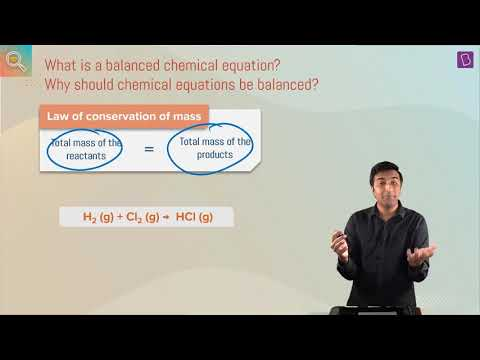#### Balancing A Chemical Equation I Class 10 I Learn With BYJU’S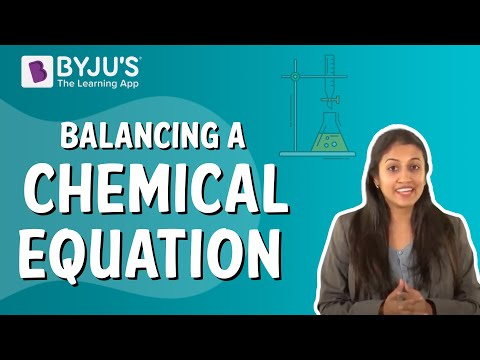#### NCERT Grade 10 Chemistry Chemical Reactions and Equations Balancing of a Chemical Reaction Q06.4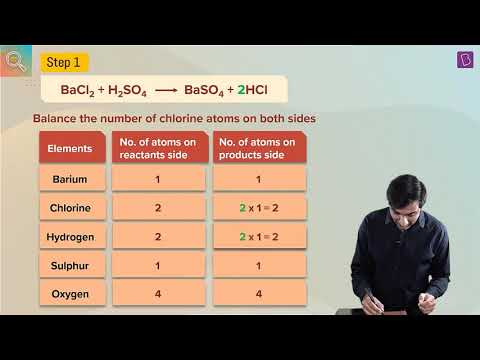#### Introduction to Chemical Bonding